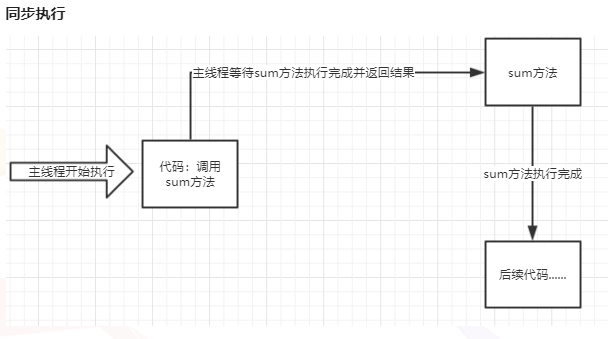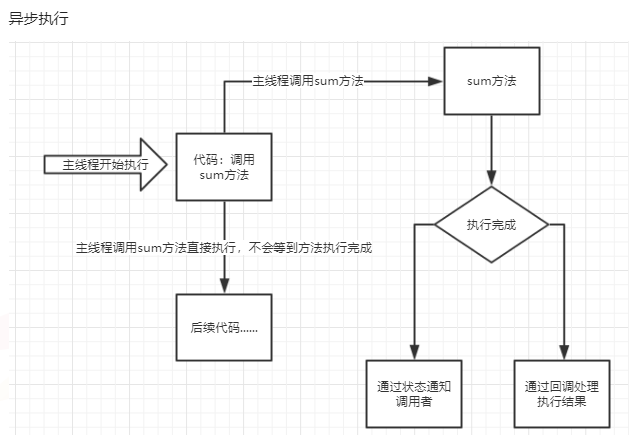*思路： 思路输入

*思路： 思路输入

1.同步和异步定义4.进程同步和进程通信

*思路： 思路输入

*思路： 思路输入

*思路： 思路输入

$\Gamma(z) = \int_0^\infty t^{z-1}e^{-t}dt,.$
$$\Gamma(z) = \int_0^\infty t^{z-1}e^{-t}dt,.$$

$$x=a_0 + \cfrac {1^2}{a_1 + \cfrac {2^2}{a_2 + \cfrac {3^2}{a_3 + \cfrac {4^2}{a_4 + …}}}}$$